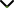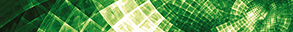# Fractional Operators in Modelling Chaotic and Real-World Problems

Publishing date
01 Jan 2022
Status
Closed
27 Aug 2021

Guest Editors

1Cheikh Anta Diop University of Dakar, Dakar, Senegal

2Consejo Nacional de Ciencia y Tecnología, Morelos, Mexico

3Federal University of Technology, Akure, Nigeria

This issue is now closed for submissions.
More articles will be published in the near future.

# Fractional Operators in Modelling Chaotic and Real-World Problems

This issue is now closed for submissions.
More articles will be published in the near future.

## DescriptionFractional calculus has become a popular topic for researchers due to its various applications in modelling physical problems, electrical circuits, chaotic systems, diffusion phenomena, epidemical models, etc. Chaotic systems are known to be sensitive to the initial conditions and the variation of the model equations' parameters. Therefore, many tools such as bifurcation maps, Lyapunov exponents, route to chaos, stability, synchronization can be used to analyze dynamics. Chaotic systems have particularly many applications in modelling electrical circuits.

Fractional calculus confirms various fractional operators with the singular kernel as the Caputo derivative and the Riemann-Liouville derivative, and the fractional operators without singular kernels as the Caputo-Fabrizio derivative and the Atangana-Baleanu derivative. There is a need to focus on the impact of the fractional-order operators (new and old) in modelling chaotic systems. Moreover, further research needs to be conducted to analyze how in a fractional context, the fractional chaotic equations can be drawn in terms of electrical circuits. The bifurcation maps and the Lyapunov exponents allow us to characterize types of chaos such as chaotic behaviour and hyperchaotic behaviour when the system's parameters vary in time. The bifurcation and the Lyapunov exponents can also be studied in a fractional context. These two algorithms detecting chaos are complex. However, they should be applicable in a fractional context. The phase portraits of the chaotic systems are also important to observe the real impact of the fractional operator's order in the dynamics of the system. For this reason, numerical algorithms are necessary due to the complexity of the equations.

The aim of this Special Issue is to bring together original research and review articles that will focus on recent advances in modelling fractional-order chaotic systems. Authors are invited to submit their last investigations in modelling chaotic systems or real-word problems with applications in fractional calculus.

Potential topics include but are not limited to the following:

• Fractional-order chaotic systems
• Numerical methods for fractional differential equations
• Numerical schemes for the recent fractional operators
• Implementation of the fractional chaotic system in modelling electrical circuits
• Stability and synchronization of the fractional-order chaotic system
• Recent advances in numerical schemes
• Mathematical modelling in fractional operators
• New fractional operators and their numerical discretization
• Analytical methods for solving fractional differential equationsJournal metrics
See full report
Acceptance rate39%
Submission to final decision44 days
Acceptance to publication22 days
CiteScore0.900
Journal Citation Indicator0.690
Impact Factor0.971Author guidelinesEditorial boardDatabases and indexing

Article of the Year Award: Outstanding research contributions of 2021, as selected by our Chief Editors. Read the winning articles.